Kinetic energy depends on ##\theta## but this argument says otherwise

• I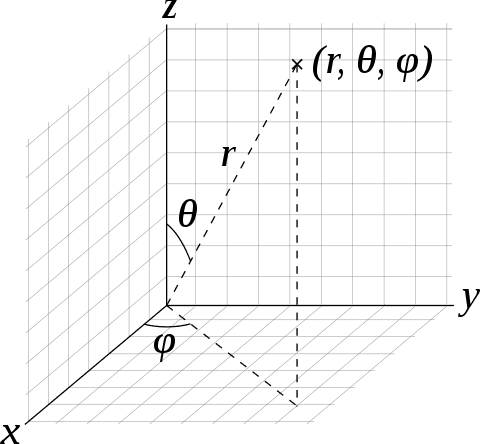A free particle with coordinates as shown has kinetic energy ##T = \frac{1}{2}m\left(\dot r^2 + r^2\dot\theta^2 + r^2\sin^2\theta\dot\phi^2\right)##
So we see ##T## depends on ##\theta##.

Now suppose we rotate our coordinate system such that only one coordinate ##\theta## changes from ##\theta## to
##\theta'## and fix it there as is shown in figure below.

In this coordinate system the kinetic energy should be the same as before since the kinetic energy should be same in all inertial frames.

However if we substitue values of ##r, \theta',\phi## in the formula for ##T## we will get a different value of ##T## hence a contradiction.

Can anyone please tell me what is wrong. Thank you.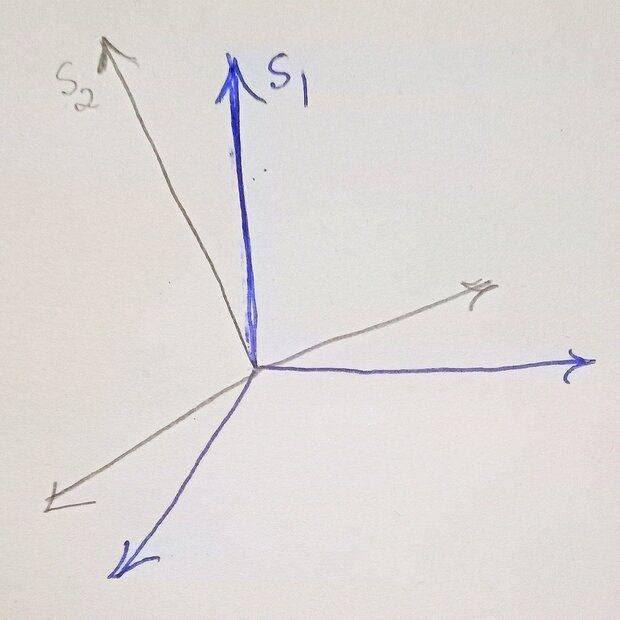Last edited:

vanhees71
Gold Member
2021 Award
I do not understand what your specific change of coordinates is. Can you give a formula?

Of course, the Hamilton principle is covariant under arbitrary changes of coordinates (diffeomorphisms). So no matter how you choose your coordinates you always describe the same physical system.

•Lnewqban
PeroK
Homework Helper
Gold Member
2021 Award
However if we substitue values of ##r, \theta',\phi## in the formula for ##T## we will get a different value of ##T## hence a contradiction.

Can anyone please tell me what is wrong. Thank you.
You didn't actually do any calculations to back up your claim! Let's see the coordinate transformation you have in mind.

•vanhees71 and Kashmir
I do not understand what your specific change of coordinates is. Can you give a formula?

Of course, the Hamilton principle is covariant under arbitrary changes of coordinates (diffeomorphisms). So no matter how you choose your coordinates you always describe the same physical system.
I don't have a formula. I'm just imagining that we rotate our coordinate system by a finite rotation with the origin fixed where it was initially in such a manner that all other variables except ##\theta## remain the same.

I hope I'm clear?

Last edited by a moderator:
You didn't actually do any calculations to back up your claim! Let's see the coordinate transformation you have in mind.
I am not able to write them in mathematics.

Lnewqban
Gold Member
I don't have a formula. I'm just imagining that we rotate our coordinate system by a finite rotation with the origin fixed where it was initially in such a manner that all other variables except ##\theta## remain the same.

I hope I'm clear?
When you rotate the coordinate system, keeping angle theta the same, the projection on x-y plane changes; therefore, at least one of the two other parameters must change.

•Dale
PeroK
Homework Helper
Gold Member
2021 Award
I am not able to write them in mathematics.
If you let ##\theta = \theta' + \alpha##, then the formula for kinetic energy becomes: $$T = \frac 1 2 m(\dot r^2 + r^2\dot\theta'^2 + r^2\sin^2(\theta' + \alpha)\dot\phi^2)$$ You can't change ##\theta## and keep the same formula as before, if that's what you are thinking.

Last edited:
•berkeman
If you let ##\theta = \theta' + \alpha##, then the formula for kinetic energy becomes: $$T = \frac 1 2 m(\dot r^2 + r^2\dot\theta^2 + r^2\sin^2(\theta' + \alpha)\dot\phi^2)$$ You can't change ##\theta## and keep the same formula as before, if that's what you are thinking.

If you let ##\theta = \theta' + \alpha##, then the formula for kinetic energy becomes: $$T = \frac 1 2 m(\dot r^2 + r^2\dot\theta^2 + r^2\sin^2(\theta' + \alpha)\dot\phi^2)$$ You can't change ##\theta## and keep the same formula as before, if that's what you are thinking.
Do you mean the correct formula is ##T = \frac 1 2 m(\dot r^2 + r^2\dot\theta^2 + r^2\sin^2(\theta' + \alpha)\dot\phi^2)## ?

since the kinetic energy should be same in all inertial frames.

... frames that are at rest with respect to each other. Without this condition your statement is not true.

... frames that are at rest with respect to each other. Without this condition your statement is not true.
Yes the two are at rest

PeroK
Homework Helper
Gold Member
2021 Award
Do you mean the correct formula is ##T = \frac 1 2 m(\dot r^2 + r^2\dot\theta^2 + r^2\sin^2(\theta' + \alpha)\dot\phi^2)## ?
Yes, if you change one of your coordinates, you must do a proper substitution in the formula. Note that with the original ##\theta## we have: $$x = r\sin \theta \cos \phi, \ y = r \sin \theta \sin \phi, \ z = r \cos \theta$$ And plugging those into $$T = \frac 1 2 m(\dot x^2 + \dot y^2 + \dot z^2)$$ gives you the formula for ##T## in terms of ##r, \theta, \phi##. That's where that formula comes from. If you choose your angles ##\theta## and/or ##\phi## differently, then you get a different formula for ##T## in terms of your new coordinates.

Yes, if you change one of your coordinates, you must do a proper substitution in the formula. Note that with the original ##\theta## we have: $$x = r\sin \theta \cos \phi, \ y = r \sin \theta \sin \phi, \ z = r \cos \theta$$ And plugging those into $$T = \frac 1 2 m(\dot x^2 + \dot y^2 + \dot z^2)$$ gives you the formula for ##T## in terms of ##r, \theta, \phi##. That's where that formula comes from. If you choose your angles ##\theta## and/or ##\phi## differently, then you get a different formula for ##T## in terms of your new coordinates.
Suppose I'm in the new system.
I'll still write $$x = r\sin \theta' \cos \phi, \ y = r \sin \theta' \sin \phi, \ z = r \cos \theta'$$ leading to
$$T = \frac{1}{2}m\left(\dot r^2 + r^2\dot\theta'^2 + r^2\sin^2\theta'\dot\phi^2\right)$$

PeroK
Homework Helper
Gold Member
2021 Award
Suppose I'm in the new system.
I'll still write $$x = r\sin \theta' \cos \phi, \ y = r \sin \theta' \sin \phi, \ z = r \cos \theta'$$ leading to
$$T = \frac{1}{2}m\left(\dot r^2 + r^2\dot\theta'^2 + r^2\sin^2\theta'\dot\phi^2\right)$$

Okay, but then ##\theta' = \theta##. That's just regular spherical coordinates.

Okay, but then ##\theta' = \theta##. That's just regular spherical coordinates.
I'll add a figure, maybe I'm not clear. Please bear with me. Thank you.

PeroK
Homework Helper
Gold Member
2021 Award
Suppose I'm in the new system.
I'll still write $$x = r\sin \theta' \cos \phi, \ y = r \sin \theta' \sin \phi, \ z = r \cos \theta'$$

The only way those three equations can hold is if you have the usual spherical coordinates, with ##\theta## measured from the positive x-axis. If you do anything different, you can't demand the same equations.

The only way those three equations can hold is if you have the usual spherical coordinates, with ##\theta## measured from the positive x-axis. If you do anything different, you can't demand the same equations.
Suppose I've rotated my coordinate system such that only ##\theta## changed to ##\theta'##. This will look like the figure shown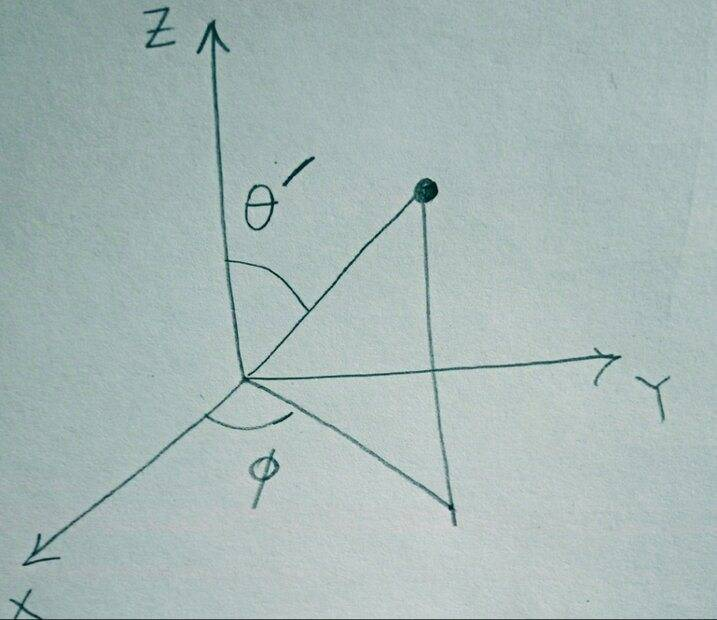Isn't it correct till now?

PeroK
Homework Helper
Gold Member
2021 Award
Suppose I've rotated my coordinate system such that only ##\theta## changed to ##\theta'##. This will look like the figure shown View attachment 290785
Isn't it correct till now?
I don't see any rotation there. I just see spherical coordinates.

I don't see any rotation there. I just see spherical coordinates.
I've just placed it upright,

I don't see any rotation there. I just see spherical coordinates.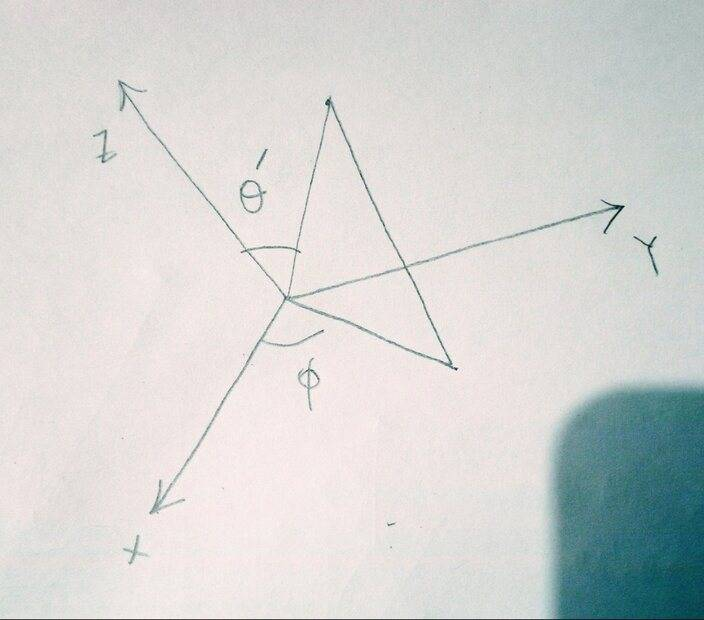PeroK
Homework Helper
Gold Member
2021 Award
So, you have a new ##z## axis?

So, you have a new ##z## axis?
Yes ,even x, y will be different. I've shown the two systems in this figure below. S2 is the rotated onekuruman
Homework Helper
Gold Member
2021 Award
Perhaps this might help. What you can always write is $$T = \frac{1}{2}m\left(\dot r^2 + r^2\dot\theta^2 + \rho^2\dot\phi^2\right)$$where ##\rho## is the projection of ##r## on the ##xy##-plane. In this form, you can see the "decomposition" of the kinetic energy into the sum of three terms, radial, meridional (if that's what it's called), and azimuthal.

•Lnewqban and PeroK
PeroK
Homework Helper
Gold Member
2021 Award
With your new ##z## axis, you no longer have $$T = \frac 1 2 m (\dot x^2 + \dot y^2 + \dot z^2)$$That is only valid for mutually orthogonal Cartesian axes. You can't change something like the z-axis without changing the formulas that involve the z-coordinate.

•Lnewqban
With your new ##z## axis, you no longer have $$T = \frac 1 2 m (\dot x^2 + \dot y^2 + \dot z^2)$$That is only valid for mutually orthogonal Cartesian axes. You can't change something like the z-axis without changing the formulas that involve the z-coordinate.
I've rotated the whole set of three axis, keeping them orthogonal during the rotation. The rotation is such that only theta changes. The mutually perpendicular axis are shown here with the only change in the coordinates of the particle being in ##\theta##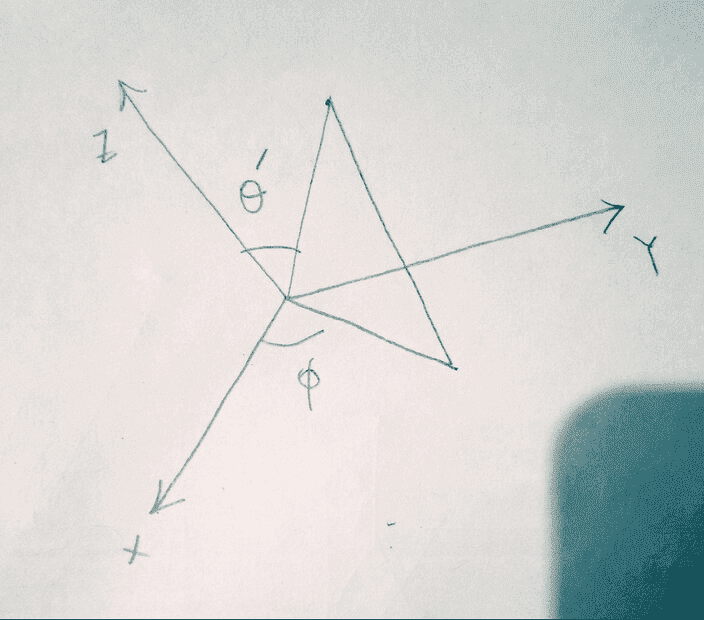PeroK
•